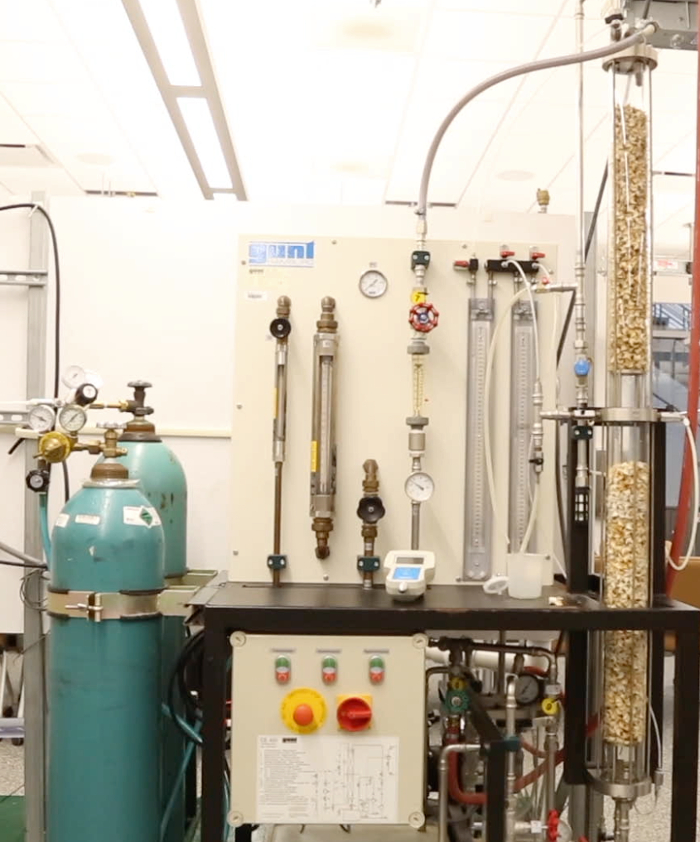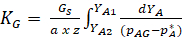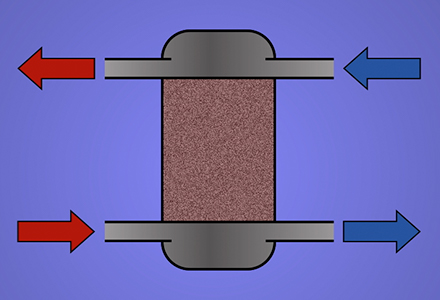Trial ends in

# Gas Absorber

### Overview

Source: Michael G. Benton and Kerry M. Dooley, Department of Chemical Engineering, Louisiana State University, Baton Rouge, LA

Gas absorbers are used to remove contaminants from gas streams. Multiple designs are used to accomplish this objective1. A packed bed column uses gas and liquid streams running counter to each other in a column packed with loose packing materials, such as ceramics, metals, and plastics, or structured packing1. The packed bed uses surface area created by the packing to create a maximum amount of efficient contact between the two phases1. The systems are low maintenance and can handle corrosive materials with high mass transfer rates1. Spray columns are another type of absorber, which uses constant direct contact between the two phases, with gas moving up and liquid being sprayed down into the gas flow1. This system only has one stage and poor mass transfer rates, but is very effective for solutes with high liquid solubility1.

The goal of this experiment is to determine how variables including gas flow rate, water flow rate, and carbon dioxide concentration affect the overall mass transfer coefficient in a gas absorber. Understanding how these parameters affect CO2 removal enables contaminant removal to be optimized. The experiment uses a randomly packed water counter-flow gas absorption column. Eight runs with two different gas flow rates, liquid flow rates, and CO2 concentrations were used. During each run, the partial pressures were taken from the bottom, middle, and top of the column unit, and the equilibrium partial pressures were calculated. These pressures were then used to find the mass transfer coefficient, and the mass transfer coefficients were compared to theoretical values.

### Principles

A gas absorption unit (Figure 1) uses contact with a liquid to remove a substance from a gas mixture. Mass is transferred from the gas mixture to the liquid via absorption.Figure 1: Typical gas absorption column.

The overall mass transfer coefficient is the rate at which the concentration of one species moves from one fluid to the other (Equation 1).(1)

In equation 1, Gs is the gas molar flow rate per cross-sectional area of the column, pAg is the partial pressure of CO2, p*A is the pressure in equilibrium with pAg, a is the interfacial area/volume or “effective area” (a function of column packing), z is the height of the packing, and KG is the overall mass transfer coefficient in mols/(pressure x interfacial area x time). Mass transfer depends on the mass transfer coefficients in each phase and the amount of interfacial area available in the absorber. Henry's Law or Raoult's Law is applied to approximate the partial pressures. They are two laws that describe the partial pressure of a component in a mixture, and are used together in order to fully describe the behavior of the mixture at the limits of the vapor-liquid equilibrium relationship. The objective of a gas absorption column is to control the effluent partial pressure of contaminant. A liquid solvent flows counter-current to the gas stream to remove the contaminant through convective mass transfer. The overall mass transfer of a water counter-flow packed column is measured in this study to determine the effects of water flow, gas flow, and CO2 gas concentration. The coefficients will then be compared to theoretical values.

### Procedure

The experiment uses a randomly packed water counter-flow gas absorption column. The column is packed with 34 cm of 13 mm berl saddles with 465 m2/m3 surface (effective) area. The pressure entering the system is about 1.42 bar with a temperature of about 26 °C, and valves at the entrance and exit of the column allow gas to escape. An "Oxy Baby" Infra-red spectrometer, directly connected to the unit at various locations, measures gas composition, and tanks of pure gas are used for calibration.

1. Operating the Gas Absorber

1. Turn on the master switch and close the adjusting valve used to control the amount of water in the column
2. Open the air flow valve completely and the adjusting valve for column pressure.
3. Set the air flow rate to the desired level (use a minimum of 20 L/min and increase as needed), and set the column pressure to ~ 1.4 bar and 25°C using the adjusting valve for pressure.
4. Start the carbon dioxide flow rate at ~ 4 L/min.
5. Set the water flow at ~ 75 L/h, and adjust the water level to maintain a constant height. Tweak if necessary while running to ensure constant height.
6. Sample the CO2 partial pressure at the base, center and head of the column using the pressure taps and the infra-red spectrometer.
7. Perform eight different runs, using two different gas flow rates, liquid flow rates, and CO2 concentrations. This will enable determination of the most important variables.
8. Allow the system to achieve steady-state when any flow rate is altered. This typically takes 30 - 45 min.

Gas absorbers are used to remove contaminants from gas streams, such as floo gas from an exhaust. A gas absorber uses a column often containing random or structured packing material. Packed bed absorbers utilize gas and liquid streams which flow counter current to each other. The contaminant gas is absorbed into the liquid stream, resulting in reduced contaminant in the exit gas. The absorption process depends heavily on the operating parameters, which must be studied in order to optimize the process. Here, we will investigate the absorption of carbon dioxide into water, and examine how the operating parameters affect the separation and efficiency of the system.

A gas absorption unit uses contact with a liquid solvent to remove the substance from a gas mixture. Mass is transferred from the gas mixture to the solvent, with the two phases near equilibrium. Then, the separation of the gas liquid phase occurs. The overall material balance for the absorber is shown here, where V and L are the vapor and liquid flow rates respectively, thus the component material balance for the absorbed component A incorporates the mole fraction of A in the vapor and liquid phase. The overall mass transfer coefficient is the rate at which the concentration of one species moves from one fluid to the other. Here, KG is the overall mass transfer coefficient, PAG is the partial pressure of the gas being absorbed, P star A is the equilibrium pressure from Henry's Law, A is the mass transfer effective area, Z is the height of the packing, and GS is the gas molar flow rate per cross sectional of the column. Mass transfer depends on the mass transfer coefficients in each phase, and the amount of interphasial area available in the absorber. Henry's Law and Raoult's Law are applied to calculate the partial pressures in equilibrium with the liquid phase concentrations. In the following experiment, a packed column gas absorber will be used to absorb carbon dioxide from a gas stream into water. The gas and water streams enter the column from the bottom and top respectively, enabling counter flow. The carbon dioxide composition at the inlet is controlled using valves for carbon dioxide and air. Then the concentration of carbon dioxide in the outlet is measured. Now that we've discussed the basics of gas absorption, let's take a look at how to run the apparatus in the lab.

The equipment used in this demonstration is a packed counterflow gas absorption column. The column is packed with 13 milliliter berl saddles at a bed depth of 34 centimeters. The valves at the entrance and exit of the column allow gas to escape, while an infrared spectrometer is used to measure the partial pressures of CO2 in the gas phase. To begin the experiment, switch on the master switch, then close the valve used to control the amount of water in the column. Open the airflow valve completely and open the adjusting valve for column pressure. Set the airflow rate to the desired level. Use a minimum of 30 liters per minute, then increase as desired. Set the column pressure to about 0.5 bar using the adjusting valve for pressure. Next, set the carbon dioxide flow rate starting at about four liters per minute, then set the water flow rate, also starting at about four liters per minute. Adjust the water flow throughout the experiment to maintain a constant water level in the tank. Sample and measure the carbon dioxide concentration as desired at the base, center, and head of the column using the in-line pressure gauges. Repeat the experiment by performing eight runs. Use two different gas flow rates, liquid flow rates, and carbon dioxide concentrations, thereby enabling the determination of the most important variables in the system. Be sure to allow the system to achieve steady state whenever a flow rate is altered.

Now that we have demonstrated how to perform the gas absorption, let's take a look at the results. First, calculate partial pressures and equilibrium partial pressures for each run, then use the partial pressures to calculate the mass transfer coefficients. The calculated values are shown here as triangles, while the predicted values, shown as the solid line, arise from computing the operating and equilibrium lines. Confidence intervals for the model values and the mean mass transfer coefficient were plotted with dashed lines. There was no deviation between the predicted and actual values, showing that the column is at steady state with equilibrium at the interface between the liquid and gas phases. Now, let's compare the mass transfer coefficients under the same operating conditions. The theoretical values, shown as the green and blue lines, showed similar trends to the experimental data. Whether the gas rate was high or low, the model and experiment behave the same, showing that the gas flow rate had little or no effect on the mass transfer coefficient in the ranges examined.

Finally, let's take a look at some applications of this technology in industry. Packed bed absorbers are the most common piece of equipment used for air pollution control. In these cases, gas absorbers are often called scrubbers. Scrubbers are used to remove corrosive fumes such as sulfuric acid, nitric acid, and hydrochloric acid from industrial gasses and vents from chemical plants, oil refineries, and pulp and paper plants. The operation of removing the absorbed gas from the solvent is called stripping. Strippers are often used in conjunction with absorbers in order to recover the absorbed gas and recycle the liquid solvent. This is especially important when the waste water contains nitrogen and phosphorus components. This waste water used to be expelled directly into oceans, however this led to the excessive growth of algae, called eutrophication, which in turn severely damaged natural ecosystems. You've just watched Jove's introduction to gas absorption.

You should now understand how a gas absorber removes an impurity from a gas stream, how to run a gas absorber in the lab, and how to analyze the data to understand the separation. Thanks for watching!

### Results

Partial pressures were taken from each trial run. Mass transfer coefficients were calculated from these and compared to predicted values (Figure 2). The predicted values arise from the calculated operating line for the absorber (see reference 2 for an in-depth discussion of the operating line). Solid lines represent the values calculated using the operating line, while triangles represent the experimental mass transfer coefficient values. Confidence intervals for the model values and the mean mass transfer coefficient were plotted with dashed lines. These values were compared to determine how the experimental parameters (liquid flow rate, gas flow rate, and CO2 partial pressure) affected the overall mass transfer coefficient. Under these operating conditions, only liquid flow rate had a statistically significant effect on mass transfer when compared to the confidence interval. The results showed that gas flow rate and feed composition had little to no effect on the mass transfer coefficient.Figure 2: Model of the predicted and actual values of the mass transfer coefficient.

Theoretical KG values for a high (30 L/min) and low (20 L/min) were calculated from mass transfer coefficient correlations and are shown as blue and green lines, respectively, in Figure 3. The experimental KG values at a variety of liquid flow rates were plotted against the theoretical values and showed similar trends, verifying the dependence of KG on liquid flow rate. The theoretical values showed some variation from the experimental values, attributable to minor experimental error.Figure 3: A graphical depiction of experimental value compared to theoretical values.

### Applications and Summary

The goal of this experiment was to use factors of gas flow rate, water flow rate, and carbon dioxide concentration to determine the overall mass transfer coefficient in a gas absorber. The experiment used a randomly packed GUNT CE 400 water counter-flow gas absorption column. Eight runs with two different gas flow rates, liquid flow rates, and CO2 concentrations were performed. Partial pressures were taken from the bottom, middle, and top of the column unit, and these pressures were then used to find the mass transfer coefficient.

Under these operating conditions, only the liquid flow rate had a significant statistical effect on mass transfer when compared to the confidence interval for the given conditions. The process is liquid-phase mass transfer controlled. Gas-related factors such as CO2 concentration and gas flow rate will have little to no significance.

Gas absorption is an important mechanism for safety in the production of chlorine3. During normal operation, gas absorbers treat any consistently occurring leaks. The start-up of a chlorine operation must be treated until it produces a gas-free product. In the event of a breakdown in the process, absorbers must be used to treat the gas that has been produced. Additionally, when new leaks form, the main emergency response unit is the standby gas absorbers. Treatment units are vitally important in these operating conditions, as they help create a safe environment when dealing with a dangerous product3.

When refining natural gas, absorption towers are used to remove natural gas liquids from the gas phase4. An absorbing oil with an affinity to natural gas liquids removes the liquid from the gas phase, purifying the product. The oil with natural gas liquids is then further purified to recover the liquids, such as butane, pentanes and other molecules. The oil can then be used again for treatment.

Absorption is also used to remove the major impurities CO2 and H2S from wellhead natural gas, converting it to pipeline gas. The process uses aqueous amines or glycols as solvents at low temperatures (typically <40 °C)5.Play Video

### Cite this

JoVE Science Education Database. Chemical Engineering. Gas Absorber. JoVE, Cambridge, MA, (2021).More

JoVE Science Education Database. Chemical Engineering. Gas Absorber. JoVE, Cambridge, MA, (2021).

Less
Copy Citation
View Video

### Get cutting-edge science videos from JoVE sent straight to your inbox every month.X